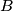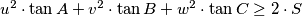### IMO Shortlist 1988 problem 27

Kvaliteta:
Avg: 0,0
Težina:
Avg: 0,0
Let$ABC$ be an acute-angled triangle. Let$L$ be any line in the plane of the triangle$ABC$. Denote by$u$,$v$,$w$ the lengths of the perpendiculars to$L$ from$A$,$B$,$C$ respectively. Prove the inequality$u^2\cdot\tan A + v^2\cdot\tan B + w^2\cdot\tan C\geq 2\cdot S$, where$S$ is the area of the triangle$ABC$. Determine the lines$L$ for which equality holds.
Izvor: Međunarodna matematička olimpijada, shortlist 1988